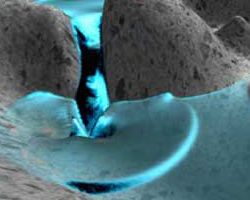delay framerate:fps
Use your fingers or mouse to control the model (hold shift key or use mouse wheel to zoom it). Canvas is matched to your browser window.

# Shallow waterThe shallow-water (or Saint-Venant) equations are     ht + ux + vy = 0,     ut + (u2/h + gh2)x + (uv/h)y = 2ghHx ,     vt + (uv/h)x + (v2/h + gh2)y = 2ghHy ,
here h(x,y, t) denotes the thickness of the water layer at point (x, y) at time t, H(x,y) is the bottom topography, (u, v) is the mass-flow of the water layer, 2g is the gravitational acceleration and t,x,y subscripts denote partial derivatives. The shallow-water equations are derived from the depth-averaged incompressible Navier-Stokes equations for the case where the surface perturbation is much smaller than the typical horizontal length scale.
The simplest possible scheme is the first-order Lax-Friedrichs scheme
Qx,y+1 = (Qx+1,y + Qx-1,y + Qx,y+1 + Qx,y-1)/4 + Δt Sx,y
+ [F(Qx+1,y) - F(Qx-1,y)]Δt/2Δx + [F(Qx,y+1) - F(Qx,y-1)]Δt/2Δy

This is a very robust scheme, which unfortunately gives excessive smearing of nonsmooth parts of the solution.

The main fragment shader is a little lengthy. You can see it in the source of this page.

 T.R. Hagen, J.M. Hjelmervik, K.-A. Lie, J.R. Natvig, M. Ofstad Henriksen
Visual simulation of shallow-water waves Simulation Modelling Practice and Theory 13 (2005) 716-726
 Miguel Lastra, Jose M. Mantas, Carlos Urena, Manuel J. Castro, Jose A. Garcia-Rodriguez
Simulation of shallow-water systems using graphics processing units
Mathematics and Computers in Simulation 80 (2009) 598-618
 Trond Runar Hagen, Martin O. Henriksen, Jon M. Hjelmervik, and Knut-Andreas Lie
How to Solve Systems of Conservation Laws Numerically Using the Graphics Processor as a High-Performance Computational Engine, 2005

Simulations on GPU
updated 4 Jan 2011